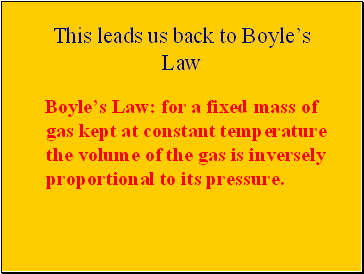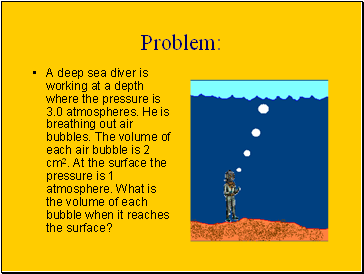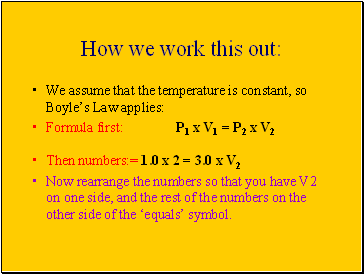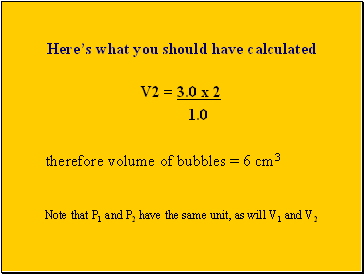# Boyle's LawPage 2

#### WATCH ALL SLIDES

Curved lines are hard to recognise, so we plot the volume against the reciprocal of pressure (ie. 1/p)

This time the points lie close to a straight line through the origin.

This means volume is directly proportional to 1/pressure or

volume is inversely proportional to pressure

Slide 11## This leads us back to Boyle’s Law

Boyle’s Law: for a fixed mass of gas kept at constant temperature the volume of the gas is inversely proportional to its pressure.

Slide 12## Problem

A deep sea diver is working at a depth where the pressure is 3.0 atmospheres. He is breathing out air bubbles. The volume of each air bubble is 2 cm2. At the surface the pressure is 1 atmosphere. What is the volume of each bubble when it reaches the surface?

Slide 13## How we work this out

We assume that the temperature is constant, so Boyle’s Law applies:

Formula first: P1 x V1 = P2 x V2

Then numbers:= 1.0 x 2 = 3.0 x V2

Now rearrange the numbers so that you have V2 on one side, and the rest of the numbers on the other side of the ‘equals’ symbol.

Slide 14Here’s what you should have calculated

V2 = 3.0 x 2

1.0

therefore volume of bubbles = 6 cm3

Note that P1 and P2 have the same unit, as will V1 and V2

Go to page:
1  2